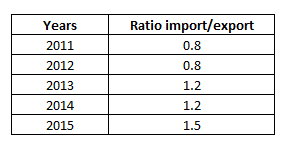# SSC CHSL 25 Jan 2017 Evening Shift

Instructions

For the following questions answer them individually

Question 91

A cylindrical vessel of height 7 cm and radius 5 cm is completely filled with sand. When this sand is poured out it forms a right circular cone of radius 8 cm what will be the height of this cone?

Question 92

What is the value of $$\frac{cosecA}{\sqrt{(cosec^2A-1)}}$$ ?

Question 93

ΔABC is right angled at B. BD is the altitude. AD is 8 cm and DC is 50 cm. What is the length of BD?

Question 94

If secA -­ tanA = x, then the value of x is

Question 95

In a rectangle

Question 96

Two fractions are such that their product is ­4 and sum is ­68/15. Find the two fractions.

Question 97

What is the total value of the stocks in lakhs rupees?

Question 98If the Imports In 2012 was Rs. 1200 crores and the total exports In the years 2012 and 2013 together was Rs. 3200 crores, then the Imports in 2013 was?

Question 99